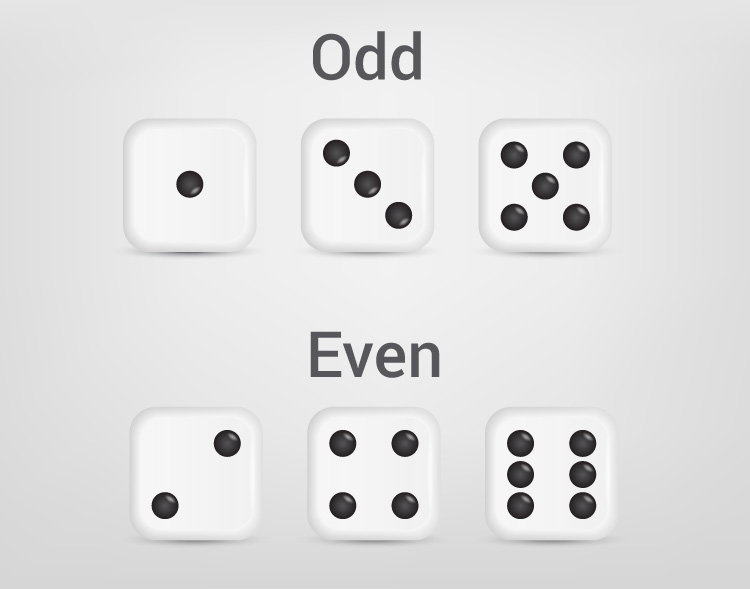# C Program to Check Whether a Number is Even or Odd

In this example, you will learn to check whether a number entered by the user is even or odd.To understand this example, you should have the knowledge of the following C programming topics:

An even number is an integer that is exactly divisible by 2. Example: 0, 8, -24

An odd number is an integer that is not exactly divisible by 2. Example: 1, 7, -11, 15

## Program to Check Even or Odd

``````#include <stdio.h>
int main() {
int num;
printf("Enter an integer: ");
scanf("%d", &num);

// True if num is perfectly divisible by 2
if(num % 2 == 0)
printf("%d is even.", num);
else
printf("%d is odd.", num);

return 0;
}
``````

Output

```Enter an integer: -7
-7 is odd.
```

In the program, the integer entered by the user is stored in the variable num.

Then, whether num is perfectly divisible by `2` or not is checked using the modulus `%` operator.

If the number is perfectly divisible by `2`, test expression `number%2 == 0` evaluates to `1` (true). This means the number is even.

However, if the test expression evaluates to `0` (false), the number is odd.

## Program to Check Odd or Even Using the Ternary Operator

``````#include <stdio.h>
int main() {
int num;
printf("Enter an integer: ");
scanf("%d", &num);

(num % 2 == 0) ? printf("%d is even.", num) : printf("%d is odd.", num);
return 0;
}

``````

Output

```Enter an integer: 33
33 is odd.
```

In the above program, we have used the ternary operator `?:` instead of the `if...else` statement.